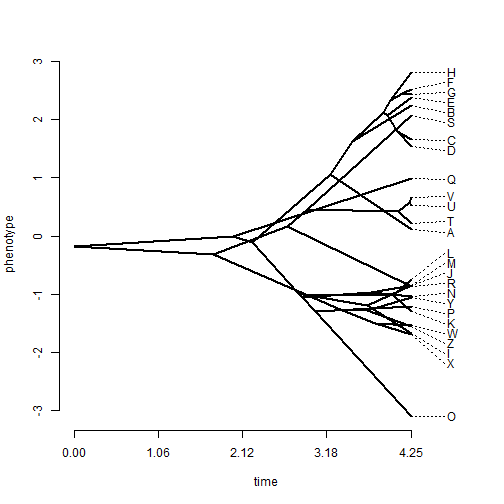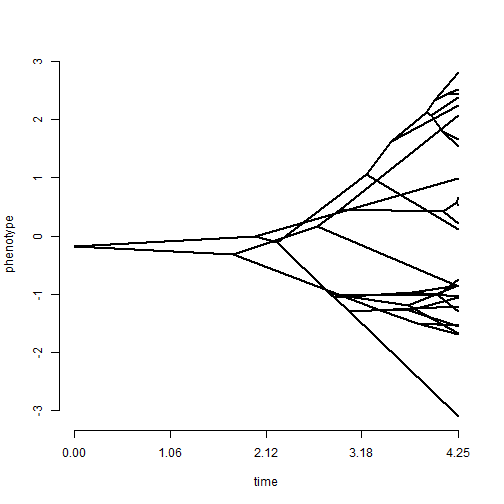## Tuesday, May 19, 2015

### Bug fix for phenogram when ftype="off"

In developing an exercise recently, I discovered a small bug that was introduced into the function `phenogram` when I changed (in the latest phytools) version the optional argument `spread.labels` to default from `FALSE` to `TRUE`. This bug causes the function to crash if labels are turned off using `ftype="off"` under the default conditions.

Here's how it manifests using simulated tree & data:

``````library(phytools)
packageVersion("phytools")
``````
``````##  '0.4.56'
``````
``````tree<-pbtree(n=26,tip.label=LETTERS)
x<-fastBM(tree)
phenogram(tree,x) ## works
````````````phenogram(tree,x,ftype="off") ## doesn't work
``````
``````## Error in optim(zz, ff, yy = yy, mo = mo, ms = ms, cost = cost, method = "L-BFGS-B", : L-BFGS-B needs finite values of 'fn'
````````````phenogram(tree,x,ftype="off",spread.labels=FALSE) ## works
``````The fix is pretty easy - I just check if `ftype="off"` and set `spread.labels=FALSE` if it is. I will put this in the next version of phytools. Here's how it works:

``````source("http://www.phytools.org/phenogram/v1.4/phenogram.R")
phenogram(tree,x)
````````````phenogram(tree,x,ftype="off") ## now works
``````OK, that's it for now.